SSC  >  SSC CPO Mock Test (Paper 1) - 4

# SSC CPO Mock Test (Paper 1) - 4

Test Description

## 200 Questions MCQ Test | SSC CPO Mock Test (Paper 1) - 4

SSC CPO Mock Test (Paper 1) - 4 for SSC 2023 is part of SSC preparation. The SSC CPO Mock Test (Paper 1) - 4 questions and answers have been prepared according to the SSC exam syllabus.The SSC CPO Mock Test (Paper 1) - 4 MCQs are made for SSC 2023 Exam. Find important definitions, questions, notes, meanings, examples, exercises, MCQs and online tests for SSC CPO Mock Test (Paper 1) - 4 below.
Solutions of SSC CPO Mock Test (Paper 1) - 4 questions in English are available as part of our course for SSC & SSC CPO Mock Test (Paper 1) - 4 solutions in Hindi for SSC course. Download more important topics, notes, lectures and mock test series for SSC Exam by signing up for free. Attempt SSC CPO Mock Test (Paper 1) - 4 | 200 questions in 120 minutes | Mock test for SSC preparation | Free important questions MCQ to study for SSC Exam | Download free PDF with solutions
 1 Crore+ students have signed up on EduRev. Have you?
SSC CPO Mock Test (Paper 1) - 4 - Question 1

### Complete the analogous pair.ACEG : JLNP :: BDFH : ?

SSC CPO Mock Test (Paper 1) - 4 - Question 2

### Re-arrange the given words in alphabetical order and tick the one that comes in the middle.

SSC CPO Mock Test (Paper 1) - 4 - Question 3

### Re-arrange the given words in alphabetical order and tick the one that comes in the middle.

SSC CPO Mock Test (Paper 1) - 4 - Question 4
Choose the word which is least like the other words in the group.
SSC CPO Mock Test (Paper 1) - 4 - Question 5
Choose the pair in which the words are differently related .
SSC CPO Mock Test (Paper 1) - 4 - Question 6
Choose the group of letters which is different from others.
SSC CPO Mock Test (Paper 1) - 4 - Question 7
Complete the analogous pair.
Warm : Hot :: ? : Anger
SSC CPO Mock Test (Paper 1) - 4 - Question 8
Complete the analogous pair.
Judges : Bench :: Teachers : ?
SSC CPO Mock Test (Paper 1) - 4 - Question 9
Complete the analogous pair.
Nose : Man : : Gill :?
SSC CPO Mock Test (Paper 1) - 4 - Question 10
Complete the analogous pair.
Court : Justice : : School : ?
SSC CPO Mock Test (Paper 1) - 4 - Question 11
A clock is so placed that at 12 noon its minute hand points towards north-east. In which direction does its hour hand point at 1.30 p.m. ?
SSC CPO Mock Test (Paper 1) - 4 - Question 12
Study the following and answer the question based on it.
1. A,B,C,D,E,F and G are sitting on a wall and they all facing to north.
2. C is at the right of D.
3. B is at the end and E is his neighbour.
4. G is sitting between E and F.
5. D is third from southen end.
Which one of the following should replace with C so that he may be third from northern?
SSC CPO Mock Test (Paper 1) - 4 - Question 13
Find the number of squares in the given figure.
SSC CPO Mock Test (Paper 1) - 4 - Question 14
Find the missing character.
Detailed Solution for SSC CPO Mock Test (Paper 1) - 4 - Question 14 The numbers in the first row form a series 12, 22, 32.
So, missing number in the first row =42=16.
The numbers in the second row form the series, 1, 2, 3, 4.
The numbers in the third row form the series 2, 4, 6.
So, missing number in the third row =6+2=8.
SSC CPO Mock Test (Paper 1) - 4 - Question 15
If the second half of the following alphabets is written in the reverse order, which will be the 10th letter to the left of 9th letter counting from the right end ?
A B C D E F G H I J K L M N O P Q R S T U V W X Y Z
SSC CPO Mock Test (Paper 1) - 4 - Question 16
Group the following figures into three classes on the basis of identical properties.
SSC CPO Mock Test (Paper 1) - 4 - Question 17
Find the missing character.
Detailed Solution for SSC CPO Mock Test (Paper 1) - 4 - Question 17 In fig. (A), ${6}^{2}=36,\phantom{\rule{0.5em}{0ex}}{8}^{2}=64,\phantom{\rule{0.5em}{0ex}}{5}^{2}=25,\phantom{\rule{0.5em}{0ex}}{7}^{2}=49.$ And, 6+8+5+7=26.
In fig. (B), ${3}^{2}=9,\phantom{\rule{0.5em}{0ex}}{5}^{2}=25,\phantom{\rule{0.5em}{0ex}}{4}^{2}=16,\phantom{\rule{0.5em}{0ex}}{9}^{2}=81.$ And, 3+5+4+9=21.
In fig. (C), ${5}^{2}=25,\phantom{\rule{0.5em}{0ex}}{12}^{2}=144,\phantom{\rule{0.5em}{0ex}}{6}^{2}=36,\phantom{\rule{0.5em}{0ex}}{8}^{2}=64.$
So, missing number = 5+12+6+8=31.
SSC CPO Mock Test (Paper 1) - 4 - Question 18
Find the missing character.
Detailed Solution for SSC CPO Mock Test (Paper 1) - 4 - Question 18 The above three numbers are multiples of the number at the bottom.
Clearly, 36, 18 and 27 are all multiples of 9.
So, the missing number is 9.
SSC CPO Mock Test (Paper 1) - 4 - Question 19
Which is the one that does not belong to the group?
SSC CPO Mock Test (Paper 1) - 4 - Question 20
Choose the odd one out.
SSC CPO Mock Test (Paper 1) - 4 - Question 21
If BINARY is coded as DHPZTX then how will KIDNAP be coded?
Detailed Solution for SSC CPO Mock Test (Paper 1) - 4 - Question 21
 B I N A R Y ↓+1 ↑ ↓+1 ↑ ↓+1 ↑ D H P Z T X

 K I D N A P ↓+1 ↑ ↓+1 ↑ ↓+1 ↑ M H F M C O
SSC CPO Mock Test (Paper 1) - 4 - Question 22
The diagram below shows group of people earning their living by various means viz.
A = By working in office
B = By working in factories
C = By running their own business

Which portion in the diagram depicts people who are earning their living from their own business as well as by working in offices?
SSC CPO Mock Test (Paper 1) - 4 - Question 23

The given diagram shows the number of students who failed in an examination comprising papers in English, Hindi and Mathematics. The total number of students who took the test is 500. What is the percentage of students who failed in atleast two subject ?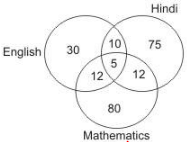Detailed Solution for SSC CPO Mock Test (Paper 1) - 4 - Question 23

Number of students who failed at least two subjects
= 10 + 5 + 12 + 12 = 39
Hence, required percentage = 39 500 x 100 = 7.8

SSC CPO Mock Test (Paper 1) - 4 - Question 24
If the first and second letters of the 'UNPRECEDENTED' are interchanged with the last and second last letters and similarly the third and the fourth letters are interchanged with the third and the fourth letters from the last respectively and so on, then what will be the 7th letter to the right of the 3rd letter from the left?
Detailed Solution for SSC CPO Mock Test (Paper 1) - 4 - Question 24 U N P R E C E D E N T E D is represented as:

Third letter from left is T and 7th letter to the right of T is R
SSC CPO Mock Test (Paper 1) - 4 - Question 25

If + means -, - means x, x means ÷ and ÷ means +, then
36 x 2+8÷ 8=?

SSC CPO Mock Test (Paper 1) - 4 - Question 26

If - means x, x means +, + means ÷ and ÷ means -, then
40 x 12 + 3 - 6 ÷ 60 = ?

Detailed Solution for SSC CPO Mock Test (Paper 1) - 4 - Question 26

Given expression : 40 + 12 ÷ 3 x 6 - 60 = 40 + 4 x 6 - 60 = 40 + 24 - 60 = 4

SSC CPO Mock Test (Paper 1) - 4 - Question 27

If 'x' means '-', '+' means '÷', '-' means '+' and '÷' means 'x' then what is the value of 136 + 4 ÷ 5 - 68 x 75?

Detailed Solution for SSC CPO Mock Test (Paper 1) - 4 - Question 27

136 ÷ 4 x 5 + 68 - 75
= 34 x 5 - 7
⇒ 170 - 7 = 163

SSC CPO Mock Test (Paper 1) - 4 - Question 28
Choose the odd one out.
SSC CPO Mock Test (Paper 1) - 4 - Question 29
Find the odd number from the given alternatives.
SSC CPO Mock Test (Paper 1) - 4 - Question 30
Choose the odd one out.
SSC CPO Mock Test (Paper 1) - 4 - Question 31
Choose the missing term out of the given alternatives.
Z, U, Q, ?, L
SSC CPO Mock Test (Paper 1) - 4 - Question 32
Find out the term in the number series which is wrong.
5, 27, 61, 122, 213, 340, 509
Detailed Solution for SSC CPO Mock Test (Paper 1) - 4 - Question 32 The terms of the series are (23 - 3), (33 - 3), (43 - 3), (53 - 3), (63 - 3), (73 - 3), (83 -3).
So, 27 is wrong and must be replaced (33 - 3) i.e. 24
SSC CPO Mock Test (Paper 1) - 4 - Question 33
Choose the missing term out of the given alternatives.
C4X, F9U, I16R, ?
SSC CPO Mock Test (Paper 1) - 4 - Question 34

Find the wrong number in the series.
2, 6, 16, 38, 84, 176, 368

Detailed Solution for SSC CPO Mock Test (Paper 1) - 4 - Question 34

2x2=4+2=6
6x2=12+4=16
16x2=32+6=38
38x2=76+8=84
84x2=168+10=178
178x2=356+12=368
.......

SSC CPO Mock Test (Paper 1) - 4 - Question 35
Find out the term in the number series which is wrong.
1, 2, 5, 10, 17, 28
SSC CPO Mock Test (Paper 1) - 4 - Question 36
Choose the correct alternative that will continue the same pattern and fill in the blank.
13, 32, 24, 43, 35, (....), 46, 65, 57, 76
SSC CPO Mock Test (Paper 1) - 4 - Question 37
Below is given statement followed by two conclusions numbered I and II. You have to consider the statement and the following conclusions and decide which of the conclusions is follows in the statement :
Statements : a. All articles are coats.
b. Some coats are tables.
Conclusions : I. Some articles are tables.
II. Some tables are articles.
SSC CPO Mock Test (Paper 1) - 4 - Question 38
Below is given statement followed by two assumptions numbered I and II. You have to consider the statement and the following assumptions and decide which of the assumptions is implicit in the statement :
Statements :
The district authority has decided to set up wireless communication along the coastline in view of the cyclonic storm hitting the coast.
Assumptions :
I. The telephone communications may be paralyzed due to the cyclonic storm.
II. The wireless communication systems may be able to withstand the fury of the cyclonic storm.
SSC CPO Mock Test (Paper 1) - 4 - Question 39
If 'red means 'white', 'white' means 'black', 'black' means 'yellow', 'yellow' means 'green' and 'green' means 'blue' and 'blue means 'indigo'; then which of the following will represent the colour of sunflower?
SSC CPO Mock Test (Paper 1) - 4 - Question 40
Bus for Chikodi village is after every hour from 7.30 am to 12.30 pm but after that it is after every one and half hours gap. One bus has gone half an hour ago, Vilas was told by a depot clerk at 5.30 pm. When was the next bus scheduled ?
SSC CPO Mock Test (Paper 1) - 4 - Question 41
In each question, five words are given. Four of them are alike in some way. One is different from them.Which is the one that does not belong to that group?
SSC CPO Mock Test (Paper 1) - 4 - Question 42
From the given alternatives, find the word which cannot be formed from the letters used in the given word.
INFRASTRUCTURE
SSC CPO Mock Test (Paper 1) - 4 - Question 43

One side of a right triangle measures 12 cm and the difference between the hypotenuse and the other side is 8 cm. The area of the triangle is

Detailed Solution for SSC CPO Mock Test (Paper 1) - 4 - Question 43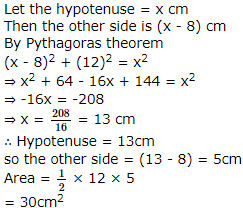SSC CPO Mock Test (Paper 1) - 4 - Question 44
Fill in the blank with appropriate word.
SSC CPO Mock Test (Paper 1) - 4 - Question 45

In the following questions, select a figure from amongst the four alternatives, which when placed in the blank space of fig. (X) would complete the pattern.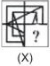SSC CPO Mock Test (Paper 1) - 4 - Question 46

In each question below is given a group of letters followed by four combinations of digits numbered (A), (B), (C) and (D). You have to find which of the combinations correctly represents the group of letters based on the following coding system and mark the number of that combination as the answer. If none of the four combinations correctly represents the group of letters, mark (E) i.e. 'None of these' as the answer.
Letter : B L C X P D S K M E
Digits :  7 1  0  8 9  3 6  2  5  4

XCMSLPK

Detailed Solution for SSC CPO Mock Test (Paper 1) - 4 - Question 46

X → 8, C → 0, M → 5, S → 6 L → 1, P → 9, K → 2 XCMSLPK → 8056192

SSC CPO Mock Test (Paper 1) - 4 - Question 47

In each question below is given a group of letters followed by four combinations of digits numbered (A), (B), (C) and (D). You have to find which of the combinations correctly represents the group of letters based on the following coding system and mark the number of that combination as the answer. If none of the four combinations correctly represents the group of letters, mark (E) i.e. 'None of these' as the answer.
Letter : B L C X P D S K M E
Digits :  7 1  0  8 9  3 6  2  5  4

KCDEBPS

SSC CPO Mock Test (Paper 1) - 4 - Question 48

Complete the analogous pair.
31. 11 : 145 :: 14 : ?

Detailed Solution for SSC CPO Mock Test (Paper 1) - 4 - Question 48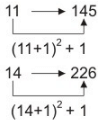SSC CPO Mock Test (Paper 1) - 4 - Question 49

Select the correct mirror image of the figure (X) from amongst the given alternatives.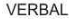SSC CPO Mock Test (Paper 1) - 4 - Question 50

Find out from amongst the four alternatives as to how the pattern would appear when the transparent sheet is folded at the dotted line.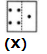SSC CPO Mock Test (Paper 1) - 4 - Question 51

Each of the following question consist of five problem figure marked 1, 2, 3, 4, 5 followed by Answer figure A, B, C, D and E. Select of figure from the answer figure which will continue the same series as given in the problem figure.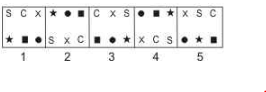SSC CPO Mock Test (Paper 1) - 4 - Question 52

In the questions, figure (X) is embedded in one of following figures. Find out the correct alternative.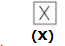SSC CPO Mock Test (Paper 1) - 4 - Question 53

Which one of the following states has a lower percentage decadal growth (1991-2001) than the other three ?

SSC CPO Mock Test (Paper 1) - 4 - Question 54

Which of the following is not included in Article 19 of the Constitution?

SSC CPO Mock Test (Paper 1) - 4 - Question 55

The Attorney-General holds office:

SSC CPO Mock Test (Paper 1) - 4 - Question 56

Can there be a common high Court for two or more States?

SSC CPO Mock Test (Paper 1) - 4 - Question 57

Which city of India has the largest Zoological garden?

SSC CPO Mock Test (Paper 1) - 4 - Question 58

Bit refers to a:

SSC CPO Mock Test (Paper 1) - 4 - Question 59

Which of the following packages is used for composition in printing press?

SSC CPO Mock Test (Paper 1) - 4 - Question 60

The Kisan Credit Card Scheme, introduced in 1998-99,is an innovative mechanism for facilitating.....:

SSC CPO Mock Test (Paper 1) - 4 - Question 61

Corporate Tax is imposed by—

SSC CPO Mock Test (Paper 1) - 4 - Question 62

What is Zero Coupon Bond?

SSC CPO Mock Test (Paper 1) - 4 - Question 63

Which of the following schemes was launched a few years back to provide wage employment to the rural people in India (The programme is being run successfully in addition to NREGA)?

SSC CPO Mock Test (Paper 1) - 4 - Question 64

By which Bill does the government purpose collection of revenues for a year?

SSC CPO Mock Test (Paper 1) - 4 - Question 65

Coal is considered as a

SSC CPO Mock Test (Paper 1) - 4 - Question 66

Compared to the rich the poor save-

SSC CPO Mock Test (Paper 1) - 4 - Question 67

Antyodaya scheme is meant to

SSC CPO Mock Test (Paper 1) - 4 - Question 68

Which temperature zone is known as the surplus zone ?

SSC CPO Mock Test (Paper 1) - 4 - Question 69

The vertical transfer of energy through the atmosphere is called :

SSC CPO Mock Test (Paper 1) - 4 - Question 70

Which is the poorest country among the following?

SSC CPO Mock Test (Paper 1) - 4 - Question 71

The length of the Sueze canal is :

SSC CPO Mock Test (Paper 1) - 4 - Question 72

Indian Naval Academy is located at;

SSC CPO Mock Test (Paper 1) - 4 - Question 73

What lead Nawab Sirajuddaulah to attack Fort William in Calcutta in 1756

SSC CPO Mock Test (Paper 1) - 4 - Question 74

The Capital of the Chola rulers during the Sangam period was-

SSC CPO Mock Test (Paper 1) - 4 - Question 75

The Swaraj Party was established in -

SSC CPO Mock Test (Paper 1) - 4 - Question 76

Who among the following was the head of the military department under Delhi Sultans ?

SSC CPO Mock Test (Paper 1) - 4 - Question 77

Who was Vrajapati during the early Vedic period

SSC CPO Mock Test (Paper 1) - 4 - Question 78

Which one of the following is NOT true about fruits ?

SSC CPO Mock Test (Paper 1) - 4 - Question 79

According to a legend Lord Krishna lifted Mount Govardhana to crush the pride of :

SSC CPO Mock Test (Paper 1) - 4 - Question 80

According to the World Health Organisation the disease which causes the death of the largest number of people today is:

SSC CPO Mock Test (Paper 1) - 4 - Question 81

Which one of the following fuels causes minimum environmental pollution?

SSC CPO Mock Test (Paper 1) - 4 - Question 82

The half-life of a radioactive element is a 5 years. The fraction the radioactive substance that remains after 20 years is

SSC CPO Mock Test (Paper 1) - 4 - Question 83

Consider the following statements about accetylene:
1. It is used in welding industry
2. It is a raw material for preparing plastics.
3. It is easily obtained by mixing silicon carbide and water.
Of these statements

SSC CPO Mock Test (Paper 1) - 4 - Question 84

An aqueous solution of copper sulphate is acidic in nature because the salt undergoes

SSC CPO Mock Test (Paper 1) - 4 - Question 85

When the same note is played on a sitar and a flute, the sound produced can be distinguished from each other because of the difference in

SSC CPO Mock Test (Paper 1) - 4 - Question 86

When water is heated from 0 C to 10 * C, its volume

SSC CPO Mock Test (Paper 1) - 4 - Question 87

The group of small pieces of rock revolving round the between the orbits of Mars and Jupiter are called

SSC CPO Mock Test (Paper 1) - 4 - Question 88

Renowned _______actress Lakshmi Devi Kanakala passed away recently.

SSC CPO Mock Test (Paper 1) - 4 - Question 89

The best known and most commonly used artificial fibre is :

SSC CPO Mock Test (Paper 1) - 4 - Question 90

A car has a speedometer to measure the :

SSC CPO Mock Test (Paper 1) - 4 - Question 91

A person in normal health requires per day :

SSC CPO Mock Test (Paper 1) - 4 - Question 92

The time period of a pendulum on moon

SSC CPO Mock Test (Paper 1) - 4 - Question 93

Anemometer measures :

SSC CPO Mock Test (Paper 1) - 4 - Question 94

Internet operations could transmit :

SSC CPO Mock Test (Paper 1) - 4 - Question 95

Which one of the following is a contagious disease ?

SSC CPO Mock Test (Paper 1) - 4 - Question 96

Which of the following machines will fail to give satisfactory result in vacuum ?

SSC CPO Mock Test (Paper 1) - 4 - Question 97

During processing, the maximum amount of food substance lost is :

SSC CPO Mock Test (Paper 1) - 4 - Question 98

India's National Cricket Championship is :

SSC CPO Mock Test (Paper 1) - 4 - Question 99

Which of the following is the best conductor of electric current ?

SSC CPO Mock Test (Paper 1) - 4 - Question 100

South Asian Association for Regional Co-operation (SAARC) was formed in:

SSC CPO Mock Test (Paper 1) - 4 - Question 101

Which country has attained the top position in the latest World FIFA Rankings?

SSC CPO Mock Test (Paper 1) - 4 - Question 102

How is the new membership of United Naitons affected?

SSC CPO Mock Test (Paper 1) - 4 - Question 103

Akbar will turn 50 when his son Jehangir turns 18. What will be Akbar's age when it will be exactly 5 times that of Jehangir?

Detailed Solution for SSC CPO Mock Test (Paper 1) - 4 - Question 103

Let us suppose when jahangir's age will be 5 times that of akabar's age will be 50 - x
Hence jahangir's age wil be 18 - x
Hence (50 - x) = 5(18 - x)
⇒ 50 - x = 90 - 5x
⇒ 4x = 40
⇒ x = 10
Hence akbar's age will be 50 - 10 or 40 years when jahangir's age was 8 years

SSC CPO Mock Test (Paper 1) - 4 - Question 104

A classroom has equal number of boys and girls.8 girls left to play Kho-Kho,leaving twice as many boys as girls in the classroom. What was the total number of girls and boys present initially?

Detailed Solution for SSC CPO Mock Test (Paper 1) - 4 - Question 104

Let number of boy and girls in class are x & y
Then, x = 2 (y - 8)
or x = 2y - 16
again, x = y
or 2y - 16 = y
or y = 16
Total Number of boys & girls are 32

SSC CPO Mock Test (Paper 1) - 4 - Question 105

The area of the quadrant of a circle whose circumference is 22 cm is

Detailed Solution for SSC CPO Mock Test (Paper 1) - 4 - Question 105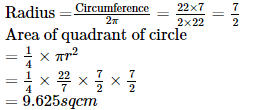SSC CPO Mock Test (Paper 1) - 4 - Question 106

A lady has only 25-paise and 50-paise coins in her purse. She has a total of 120 coins and total amount is Rs 50. The number of coins of each type in her purse is respectively

SSC CPO Mock Test (Paper 1) - 4 - Question 107

Given that x+2 and x-3 are factors of x3 + ax + b, the values of a and b are

Detailed Solution for SSC CPO Mock Test (Paper 1) - 4 - Question 107

Let p(x) = x3 + ax + b
x + 2 is a factor of p(x)
So, p(-2) = 0
(-2)3 + a(-2) + b = 0
⇒ - 8 - 2a + b = 0
⇒ b - 2a - 8 = 0 .....(1)
x - 3 is a factor of p(x)
So, p(3) = 0
(3)3 + a(3) + b = 0
⇒ 27 + 3a + b = 0 .....(2)
Subtract equation (1) from (2) we get 5a + 35 = 0
⇒ 5a = - 35
⇒ a = -7
b = -6

SSC CPO Mock Test (Paper 1) - 4 - Question 108

If x = 1 2 (√3 + 1), then the value of 4x3 + 2x2 - 8x + 7 is-

Detailed Solution for SSC CPO Mock Test (Paper 1) - 4 - Question 108

If x = 1 2 (√3 + 1)
⇒ (2x - 1)2 = 3
⇒2x2 - 2x - 1 = 0
∴ 4x3 + 2x2 - 8x + 7
= 2x (2x2 - 2x - 1) + 3 (2x2 - 2x - 1) + 10
= 2x (0) + 3 (0) + 10 = 10

SSC CPO Mock Test (Paper 1) - 4 - Question 109

A rectangular park is 200 by 150m. Two cross paths are to be made one parallel to the length and the other parallel to the breadth. The longer path is to be 3 m wide and the shorter is to be 2 m wide. The cost of grassing the remaining part of the park @ Rs. 1000 per ha is

Detailed Solution for SSC CPO Mock Test (Paper 1) - 4 - Question 109

Area of the park minus the paths
= (200 - 3) (150 - 2)
= 197 x 147 sq. m
= 29156 sq. m
The cost of grashing the remaming part of the park = Rs 29156 10000 x 1000
= Rs 2915.6

SSC CPO Mock Test (Paper 1) - 4 - Question 110

The average weight of 16 tall boys in a class is 50.25 kg and that of the remaining 8 boys is 45.15 kg. Find the average weight of all boys in the class.

SSC CPO Mock Test (Paper 1) - 4 - Question 111

If the lines x - y - 1 = 0, 4x + 3y = K and 2x - 3y + 1 = 0 are concurrent, then K is

SSC CPO Mock Test (Paper 1) - 4 - Question 112

A sum of money doubles itself at compound interest in 15 years. In how many years will it become eight times?

SSC CPO Mock Test (Paper 1) - 4 - Question 113

The average annual income (in Rs.) of certain argicultural workers is S and that of other workers is T. The number of agricultural workers is 11 times that of other workers. Then the average monthly income (in Rs.) of all the workers is:

Detailed Solution for SSC CPO Mock Test (Paper 1) - 4 - Question 113

Let the number of other workers be x
Then, number of agricultural workers = 11x
Total number of workers = 12x
∴ Average monthly income = S x 11x + T x x /12x = 11S + T/ 12

SSC CPO Mock Test (Paper 1) - 4 - Question 114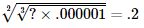SSC CPO Mock Test (Paper 1) - 4 - Question 115

By selling an umberella for Rs 30,a shopkeeper gains 20%. During a clearance sale,the shopkeeper allows a discount of 10% on the marked price. His gain % during sale is

SSC CPO Mock Test (Paper 1) - 4 - Question 116

Which of the following is equal to 3.14x106?

SSC CPO Mock Test (Paper 1) - 4 - Question 117

The orthocentre of a triangle divides the medians in the ratio :

SSC CPO Mock Test (Paper 1) - 4 - Question 118

D is the mid-point of side BC of ∆ABC and O is a point on AD. Then, the correct statement out of the following is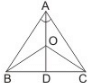Detailed Solution for SSC CPO Mock Test (Paper 1) - 4 - Question 118

AD is a median of ∆ABC.
Hence, ar. ∆ABD = ar. ∆ACD.
Also, OD is a median of ∆BOC. Hence,ar. ∆OBD = ar. ∆OCD.
Subtracting the second result from the first we obtain the result.

SSC CPO Mock Test (Paper 1) - 4 - Question 119

In the given figure AB || CD and EF || GH. If x = 80, then y = ....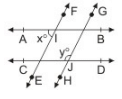SSC CPO Mock Test (Paper 1) - 4 - Question 120

AB∥CD and P is a point between the lines, the measure of the angles ∠ABP+∠BPD+∠CDP is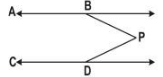SSC CPO Mock Test (Paper 1) - 4 - Question 121

The smallest three-digit prime number is

SSC CPO Mock Test (Paper 1) - 4 - Question 122

A balloon,whose radius is r,subtends an angle α at the eye of an observer when the angle of elevation of its centre is β.The height of its centre is

SSC CPO Mock Test (Paper 1) - 4 - Question 123

4598 is 95% of

SSC CPO Mock Test (Paper 1) - 4 - Question 124

The ratio of males and females in a city is 7:8 and the percentage of children among males and females is 25% and 20% respectively. If the number of adult females in the city is 156800 What is the total population?

SSC CPO Mock Test (Paper 1) - 4 - Question 125

The HCF of three numbers is 24. If they are in the ratio 35:55:77,then the numbers are

SSC CPO Mock Test (Paper 1) - 4 - Question 126

A watch is sold for Rs 880 at a loss of 20%. For how much should it be sold to gain 10%?

SSC CPO Mock Test (Paper 1) - 4 - Question 127

If 5% more is gained by selling an article for Rs 350 than by selling it for Rs 340, the cost of the article (in rupees) is

Detailed Solution for SSC CPO Mock Test (Paper 1) - 4 - Question 127

Let Cost price of an article = Rs x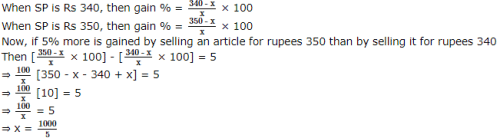⇒ x = Rs 200 = C.P. Of an article

SSC CPO Mock Test (Paper 1) - 4 - Question 128

The value of 0.234 is

SSC CPO Mock Test (Paper 1) - 4 - Question 129

Two numbers are respectively 20% and 50% more than a third number. The ratio of the two numbers is :

SSC CPO Mock Test (Paper 1) - 4 - Question 130

A man ordered 4 pairs of black socks and some pair of brown socks. The price of a black pair is double that of a brown pair. While preparing the bill,the clerk interchanged the number of black and brown pairs by mistake which increased the bill by 50%. The ratio of the number of black and brown pairs of socks in the original order was

Detailed Solution for SSC CPO Mock Test (Paper 1) - 4 - Question 130

Let x pairs of brown socks were ordered
Let P be the price of a brown pair
Therefore price of black pair of sock = 2P
Then 4P + 2Px = 1.5 (xP + 8P)
⇒ 4P + 2Px = 3 2 (xP + 8P)
⇒ 8P + 4Px = 3xP + 24P
⇒ Px = 16P or x = 16
The ratio of the number of black and brown pairs of socks in the original order was 1:4

SSC CPO Mock Test (Paper 1) - 4 - Question 131

If the sides of a triangle are 3,5,7, it has

SSC CPO Mock Test (Paper 1) - 4 - Question 132

A sum invested at 5% simple interest per annum grows to Rs. 504 in 4 years. The same amount at 10% simple interest per annum in 2.5 years will grow to :

SSC CPO Mock Test (Paper 1) - 4 - Question 133

If 2∗5 is a perfect square, which of the following digits can replace the ∗ ?

SSC CPO Mock Test (Paper 1) - 4 - Question 134

A milk tanker cylindrical is shape having diameter 2 m and length 4.2 m supplies milk to two booths A and B in the ratio 3:2. Milk booth A is a rectangular vessel having base area as 3.96m2 and milk booth B is a cylindrical vessel having diameter 2m. The level of milk in vessels in booths A and B respectively are

SSC CPO Mock Test (Paper 1) - 4 - Question 135

sin50º-sin70º+sin10º=

SSC CPO Mock Test (Paper 1) - 4 - Question 136

If cosθ=(3/5) and cosφ=(4/5), where θ and φ are positive acute angles then cos((θ-φ)/2)=

SSC CPO Mock Test (Paper 1) - 4 - Question 137

The value of cosA-sinA when A=(5π/4), is

SSC CPO Mock Test (Paper 1) - 4 - Question 138

A thief steals a car at 2.30 p.m. and drives it at 60 kmph. The theft is discovered at 3 p.m. and the owner sets off in another car at 75 kmph. When will he overtake the thief?

SSC CPO Mock Test (Paper 1) - 4 - Question 139

X and Y can do a piece of work in 20 days and 12 days respectively. X started the work alone and then after 4 days Y joined him till the completion of the work. How long did the work last?

SSC CPO Mock Test (Paper 1) - 4 - Question 140

The number of coins of radius 0.75 cm and thickness 0.2 cm to be melted to make a right circular cylinder of height 8 cm and base radius 3 cm is :

SSC CPO Mock Test (Paper 1) - 4 - Question 141

Find the minimum number of cubes required to construct a cuboid of dimension 20cm x 16cm x 12cm ?

Detailed Solution for SSC CPO Mock Test (Paper 1) - 4 - Question 141

Volume of cuboid = 20 x 16 x 12 = 3840 cm3
Since minimum no. of cubes required
So length of cube = HCF of 20, 16, 12 cm = 4
Volume of each cube = (4)3 = 64cm3
minimum no of cubes = Vol. of cuboid Vol. of cube = 3840 64 = 60

SSC CPO Mock Test (Paper 1) - 4 - Question 142

Study the following graph carefully and answer the questions given below.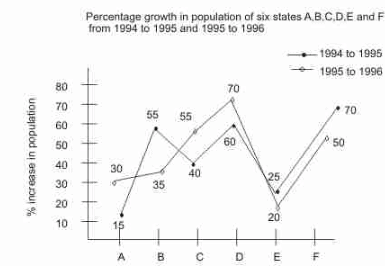Population of State 'F' in 1995 approximately what percent of its population in 1996 ?

Detailed Solution for SSC CPO Mock Test (Paper 1) - 4 - Question 142

Required percentage = × 100% = 66.66% ≈ 67% [Let population in 1995 be 100 then population in 1996 is 150]

SSC CPO Mock Test (Paper 1) - 4 - Question 143

Study the following graph carefully and answer the questions given below.If the popualtion of State 'B' in the year 1994 was 5 lakh, what was approximately its population in the year 1996 ?

SSC CPO Mock Test (Paper 1) - 4 - Question 144

Study the following graph carefully and answer the questions given below.If the population of states C and D in 1995 are in the ratio 2 : 3 respectively and the population of states 'C' in 1994 was 2.5 lakh, what was the population of State 'D' in 1995 ?

SSC CPO Mock Test (Paper 1) - 4 - Question 145

Study the following graph carefully and answer the questions given below.In 1994 population of states B and D are equal and the population of States B in 1996 is 20.925 lakhs. What approximate was the population of State 'D' in 1996 ?

Detailed Solution for SSC CPO Mock Test (Paper 1) - 4 - Question 145

Let population of B in 1994 be x then x × 1.55 × 1.35 = 20.925 lakh ⇒ x = 10 lakh An in 1994 population D = population of B, Hence population of D in 1994 is 10 lakh ∴ Population of D in 1996 is = 10 × 1.6 × 1.7 lakh = 27.2 lakh ≈ 27 lakh

SSC CPO Mock Test (Paper 1) - 4 - Question 146

Study the following graphs carefully to answer the questions given below it.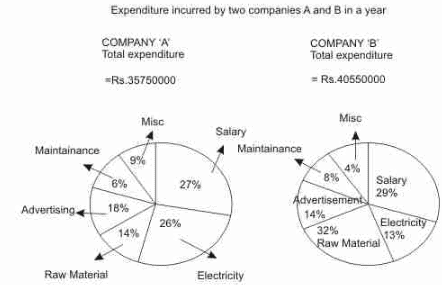What is the amount (in Rs.) spent by Company 'A' on Electricity ?

SSC CPO Mock Test (Paper 1) - 4 - Question 147

Study the following graphs carefully to answer the questions given below it.How much (in Rs.) have both companies together spent on raw material ?

Detailed Solution for SSC CPO Mock Test (Paper 1) - 4 - Question 147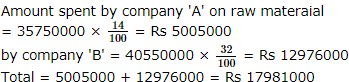SSC CPO Mock Test (Paper 1) - 4 - Question 148

Study the following graphs carefully to answer the questions given below it.What is the ratio of amount spent by Company A on Advertisement and Electricity respectively ?

Detailed Solution for SSC CPO Mock Test (Paper 1) - 4 - Question 148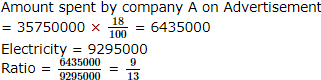SSC CPO Mock Test (Paper 1) - 4 - Question 149

Study the following graphs carefully to answer the questions given below it.On which item is there least expenditure ?(irrespective of Company A or B)

Detailed Solution for SSC CPO Mock Test (Paper 1) - 4 - Question 149

Company 'A' expenditure on miscellaneous = 35750000 × 9/100= Rs 3217500 company 'B' = 40550000 × 4/100 = Rs 1622000 So least expenditure is in miscellaneous of company 'B'

SSC CPO Mock Test (Paper 1) - 4 - Question 150

Study the following graphs carefully to answer the questions given below it.What is the total amount (in Rs.) spent by Company 'B' on Raw Material, Maintainance and salary together ?

Detailed Solution for SSC CPO Mock Test (Paper 1) - 4 - Question 150

Company 'B' spend on Raw material = 40550000 × 32/100= 12976000
maint ainance = 40550000 × 8/100= 3244000
salary = 40550000 ×29/100 = 11759500 Total = Rs 27979500

SSC CPO Mock Test (Paper 1) - 4 - Question 151

Two pipes can fill a cistern in 3 hrs and 4 hrs respectively and a waste pipe can empty it in 2 hrs. If all the three pipes are kept open, then the cistern will be filled in

SSC CPO Mock Test (Paper 1) - 4 - Question 152

Find the antonym of AUTHENTIC.

SSC CPO Mock Test (Paper 1) - 4 - Question 153

Find the Antonym of HOSTILE.

SSC CPO Mock Test (Paper 1) - 4 - Question 154

Find the antonym of DISPARAGE

SSC CPO Mock Test (Paper 1) - 4 - Question 155

Find the Antonym of FLAGITIOUS

SSC CPO Mock Test (Paper 1) - 4 - Question 156

Choose the correctly spelt word.

SSC CPO Mock Test (Paper 1) - 4 - Question 157

Choose the correctly spelt word.

SSC CPO Mock Test (Paper 1) - 4 - Question 158

SSC CPO Mock Test (Paper 1) - 4 - Question 159

Choose the correctly spelt word.

SSC CPO Mock Test (Paper 1) - 4 - Question 160

Choose the correctly spelt word.

SSC CPO Mock Test (Paper 1) - 4 - Question 161

Choose the correctly spelt word.

SSC CPO Mock Test (Paper 1) - 4 - Question 162

Fill in the blank with appropriate word.
I congratulate you ........ your success.

SSC CPO Mock Test (Paper 1) - 4 - Question 163

Fill in the blank with appropriate word.
He was in trouble because he did not.......the instructions.

SSC CPO Mock Test (Paper 1) - 4 - Question 164

Fill in the blank with appropriate word.
The passengers and crew members of the aeroplane had a.......escape when it was taking off from the runway.

SSC CPO Mock Test (Paper 1) - 4 - Question 165

Fill in the blank with appropriate word.
Your case is so unique that I am not getting any.........to support it.

SSC CPO Mock Test (Paper 1) - 4 - Question 166

Choose the one which can be substituted for the given words/sentences :
Extreme old age when a man behaves like a fool

SSC CPO Mock Test (Paper 1) - 4 - Question 167

Choose the one which can be substituted for the given words/sentences :
Thing that can be felt or touched

SSC CPO Mock Test (Paper 1) - 4 - Question 168

Choose the one which can be substituted for the given words/sentences :
To speak to oneself.

SSC CPO Mock Test (Paper 1) - 4 - Question 169

Spot the error.

SSC CPO Mock Test (Paper 1) - 4 - Question 170

spot the error.

SSC CPO Mock Test (Paper 1) - 4 - Question 171